PrecisionCalc
xl
Precision

(When xlPrecision is installed, this tutorial is also available from within Excel by choosing Tools | xlPrecision | xlPrecision Help and Function Reference.)

Tutorial

(This is a detailed, in-depth tutorial. For a quicker start, you may prefer to begin with the Quick-Start Tutorial.)

The first step in learning to use xlPrecision is to understand the limitations in Excel that xlPrecision solves. This tutorial starts by walking you through those limitations, with simple examples showing how xlPrecision solves them.

Then, in the Using xlPrecision section, it goes into more detail on getting the most from xlPrecision.

## Excel Limitation #1:  15 Significant Digits Excel's formulas often round their results.

• Create a new workbook in Excel.

• In cell A1, enter "67" (without quotes).

• In cell A2, enter "89" (without quotes).

• In cell A3, enter "=A1/A2" (without quotes).

• Right-click cell A3 and choose Format Cells.

• On the Number tab, in the Category listbox, select Number.

• In the Decimal Places spinner, enter the maximum (30), and click OK.

• Widen column A as much as necessary to see the number.

• Note that cell A3 shows:
0.752808988764045000000000000000

The correct answer would continue infinitely. Excel returns only 15 significant digits.

(Significant digits is the quantity of digits from the left-most non-zero digit to the right-most non-zero digit, regardless of the location of the decimal point.)

To see how xlPrecision solves that limitation:

• In cell A4, enter this xlPrecision formula:

• =xlpDIVIDE(A1,A2)
• Note that xlPrecision returns this in cell A5:
• 0.752808988764044943820224719101123595505617977528089887640449438202247191011235955056179775280898876404

• For more details on using xlPrecision, skip down to the Using xlPrecision section below.

## Excel Limitation #2:  Excel Automatically Rounds Your Numbers When you enter a number in Excel, Excel often permanently changes some of the digits to zero.

• Create a new workbook in Excel.

• In cell A1, enter:

• 123456789123456789

• Look in the formula bar. Note that Excel has truncated it to:

• 123456789123456000

• Note that Excel didn't even round your number correctly; instead Excel simply replaced all digits after the 15th digit to a zeros.

• In cell A3, enter:

• '123456789123456789

• Be sure to include the leading apostrophe.

•  Note that this time Excel did not change anything. However, watch what happens when you try to do any arithmetic with that number:

• In cell A4, enter:

• =A3+0
• If it returns the error "#VALUE!":

• Click on cell A1, press F2 to activate the formula bar, and then press Enter.

• Then do the same on cell A3 if necessary.

• Right-click cell A4, and select Format Cells. On the Number tab, select the Number category. Change Decimal Places to 0. Then click OK.

• Note that Excel returns this in cell A4:

• 123456789123456000

• All you did was try to add 0, and Excel truncated it to 15 digits!

To see how xlPrecision solves that limitation:

• In cell A5, enter this xlPrecision formula:

• Note that xlPrecision returns this in cell A5:
• 123456789123456789.1

• For more details on using xlPrecision, skip down to the Using xlPrecision section below.

## Excel Limitation #3:  Binary Conversion Errors Even with small numbers, Excel's formulas often return inexact results.

• Create a new workbook in Excel.

• In cell A1, enter "1000.8" (without quotes).

• In cell A2, enter "1000" (without quotes).

• In cell A3, enter "=A1-A2" (without quotes).

• Note that cell A3 shows "0.8", which is correct. But things are not as they seem. Excel is showing a slightly different number than it is actually storing in cell A3. To see what number Excel is actually storing in cell A3, continue with the following steps.

• Right-click cell A3 and choose Format Cells.

• On the Number tab, in the Category listbox, select Number.

• In the Decimal places spinner, increase decimal places to at least 15. Click OK.

• Note that cell A3 now shows:

• 0.799999999999955

0.799999999999955 is the number Excel is actually storing in A3, and that is the number that any formula referencing cell A3 will be given to use. It's very close to 0.8. But with enough repetition, as with summing a large range of numbers that each have a similar small inaccuracy, the total discrepancy can become large.

To see how xlPrecision solves that limitation:

• In cell A4, enter this xlPrecision formula:

• =xlpSUBTRACT(A1,A2)
• Note that xlPrecision returns this in cell A5:
• 0.8

• For more details on using xlPrecision, skip down to the Using xlPrecision section below.

## Excel Limitation #4:  Very Large & Very Small Numbers Excel doesn't understand numbers that are very large or very close to zero.

• Create a new workbook in Excel.

• In cell A1, enter "9E+307" (without quotes).

• In cell A2, enter "1" (without quotes).

• In cell A3, enter "=A1*A2" (without quotes).

• Note that cell A3 returns "9.00E+307", indicating that the operation was successful. This shows that Excel is able to work with numbers as large as 9E+307 (9 with 307 zeroes after it).

• Now, change cell A2 from "1" to "10" (without quotes).

• Note that cell A3 now returns the error value "#NUM!". This shows that Excel is unable to work with numbers as large as 9E+308 (9 with 308 zeroes after it).

To see how xlPrecision solves that limitation:

• In cell A4, enter this xlPrecision formula:

• =xlpMULTIPLY(A1,A2,,,,TRUE)
• Note that xlPrecision returns this in cell A5:
• 9.0E+308

• For more details on using xlPrecision, skip down to the Using xlPrecision section below.

The same is true -- except worse! -- for numbers that are very close to zero:

• In cell B1, enter "1E-307" (without quotes).

• In cell B2, enter "1" (without quotes).

• In cell B3, enter "=B1/B2" (without quotes).

• Note that cell A3 returns "1.00E-307", indicating that the operation was successful. This shows that Excel is able to work with numbers as small as 1E-307 (a decimal point, then 306 zeroes, then a 1).

• Now, change cell B2 from "1" to "10" (without quotes).

• Note that cell B3 now returns:

• 0.00E+00

• This indicates that as far as Excel is concerned, 1E-308 is actually equal to zero! It would be far better for Excel to return the error value "#NUM!", correctly indicating that it is unable to work with numbers that small.

• Note that no matter how large you make the number in cell B2, B3 continues to return "0.00E+00", indicating that Excel still considers it equal to zero.

To see how xlPrecision solves that limitation:

• In cell A4, enter this xlPrecision formula:

• =xlpDIVIDE(B1,B2,,,,TRUE)
• Note that xlPrecision returns this in cell A5:
• 1.0E-308

## Using xlPrecision

xlPrecision provides high precision in Excel by replacing Excel's low precision arithmetic operators (+, - *, /, ^), comparison operators, (>, >=, <, <=, =), and some of its worksheet functions (SUM, MEDIAN, RANK, and many others), with custom worksheet functions, such as xlpADD, xlpMULTIPLY, xlpISLESS, xlpMEDIAN, etc. For a complete list of xlPrecision functions, see the Function Reference.

Unfortunately, Excel does not allow changing the behavior of its built-in operators and worksheet functions, so it is not possible for xlPrecision to apply high precision to your existing worksheet formulas. Using xlPrecision requires modifying your worksheet formulas by replacing Excel's built-in operators with xlPrecision's custom worksheet functions. Of course, you only have to modify those formulas from which you want high precision.

For example, to get high precision from this worksheet formula:

=2/3

Change the formula to this:

=xlpDIVIDE(2,3)

Be sure to put a comma (",") between the 2 and the 3, not a slash ("/").

 Depending on your world region and language, you might need to use a different character than the comma. Use whatever character you normally use to separate arguments in Excel functions.  In many locales, that character is a semi-colon (;). If you're not sure, go into Windows' Regional/Language Options Control Panel and find your "List Separator" character. Use that character wherever xlPrecision documentation shows a comma. This example shows that the list separator character for French is the semi-colon: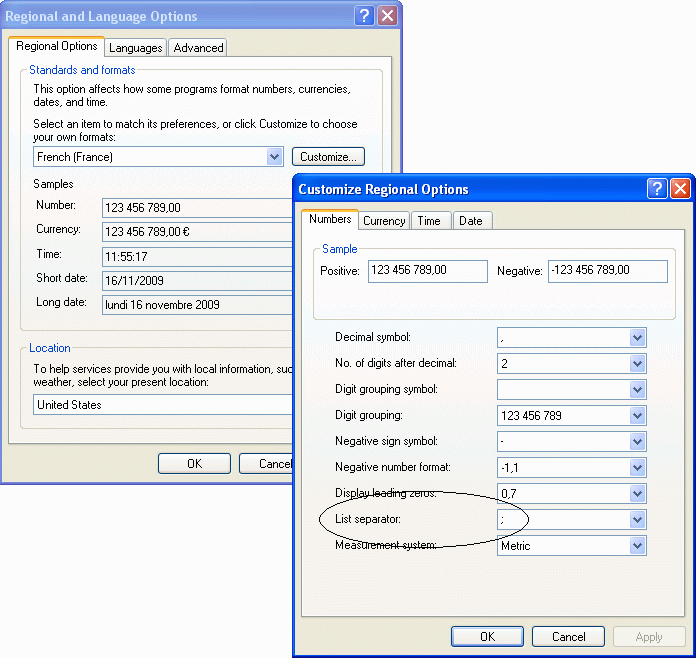The result should be as long as your edition of xlPrecision allows, or the default length of 100 significant digits, whichever is shorter. For example, if you are using the 25SD edition, the result should be 25 significant digits long, like this:

0.6666666666666666666666667

xlPrecision results are returned as text that look like numbers, not as values that Excel recognizes as numbers. This is because Excel would immediately truncate the results to 15 significant digits if it recognized them as numbers.

You can use the results of xlPrecision formulas as the operands in other xlPrecision formulas, but using them as operands in Excel's arithmetic functions will truncate them to 15 significant digits.

If you have a complex formula with more than one arithmetic operator or comparison operator, you may need to replace them all with xlPrecision functions. For example, in this formula, Excel discards the high precision returned by xlpDIVIDE when it evaluates the subtraction with Excel's built-in operator, the minus sign:

=xlpDIVIDE(A1,B1)-C1

To maintain high precision in that example, replace the minus sign with xlpSUBTRACT:

=xlpSUBTRACT(xlpDIVIDE(A1,B1),C1)

## Avoid Long Recalculations

When returning numbers with very high significant digits, or with very large operands, xlPrecision can take a long time to calculate. To prevent Excel from unnecessarily recalculating them, convert the affected formulas to text by copying them and then Paste Special > Values. And then be sure to save the workbook before closing it.

## Specifying Quantity of Significant Digits

The number of significant digits xlPrecision provides depends on the edition of xlPrecision you have. For example, the free edition provides 500 significant digits. Paid versions provide up to 32,767 significant digits. To determine how many significant digits your edition of xlPrecision provides, go to Excel's Tools menu and choose xlPrecision | About xlPrecision. One of the lines on the About box that pops up starts with "SD Edition:" and indicates the maximum number of significant digits your edition of xlPrecision provides.

Another way to determine how many significant digits your edition of xlPrecision provides is to enter "=xlpSD()" in any cell (without quotes, and xlpSD is not case-sensitive). The number returned is the maximum number of significant digits your edition of xlPrecision provides.

If you don't specify how many significant digits you want, you'll get a maximum of 100, to prevent inadvertently starting long calculations. You can customize that maximum in the About box: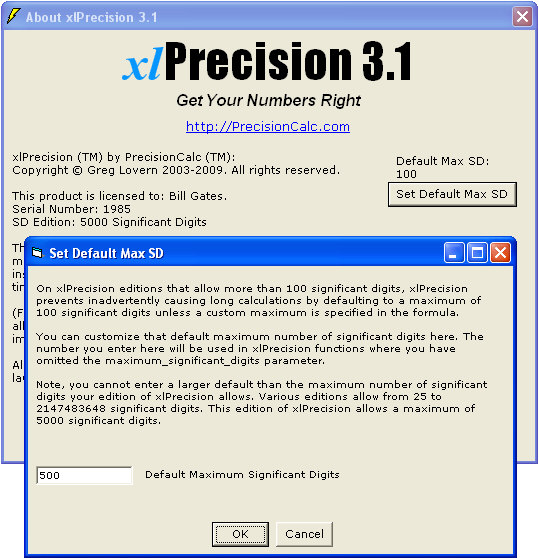For each xlPrecision function, you can specify any number of significant digits up to 32,767. For example, to get 1,000 significant digits, change the formula to this:

=xlpDIVIDE(2,3,,,,,1000)

If you specify more significant digits than your xlPrecision edition allows, then you'll get the maximum allowed by your edition.

Alternatively, you can specify fewer significant digits to speed up calculation.

Depending on how many significant digits your xlPrecision formula returns, the result may be too long to conveniently view. You can view the full result by right-clicking the cell and choosing Format Cells | Alignment | Wrap Text, and widening the column to the width of the screen. To view the full result without changing column widths or wrapping text, copy the cell and paste into Notepad or a word processor.

## Other Arithmetic Operators

xlpADD(), xlpSUBTRACT(), xlpMULTIPLY(), xlpROOT(), and xlpPOWER() all work essentially the same way as xlpDIVIDE():

 Change this: To this: =2+3 =xlpADD(2,3) =2-3 =xlpSUBTRACT(2,3) =2*3 =xlpMULTIPLY(2,3) =2/3 =xlpDIVIDE(2,3) =2^(1/3) =xlpROOT(2,3) =2^3 =xlpPOWER(2,3)

The comparison operators are similar too:

 Change this: To this: =2=3 =xlpISEQUAL(2,3) =2<>3 =xlpISNOTEQUAL(2,3) =2>3 =xlpISGREATER(2,3) =2>=3 =xlpISGREATEROREQUAL(2,3) =2<3 =xlpISLESS(2,3) =2<=3 =xlpISLESSOREQUAL(2,3)

If any of the xlPrecision functions return "#VALUE!", make sure you are separating the operands with a comma, not an arithmetic operator. For example, if you enter "=xlpADD(A1+A2)" instead of "=xlpADD(A1,A2)", it will return "#VALUE!".

For a complete list of xlPrecision functions, along with detailed information on using them and examples, see the Function Reference.

## Long Operands

So far in this tutorial, the numbers in cells A1 and A2 are entered in Excel as numbers. But if you use operands longer than 15 significant digits, Excel will truncate them to 15 significant digits. For example:

• In cell A1, change the number 67 to 12345678901234567890.

• Right-click cell A1 and choose Format Cells.

• On the Number tab, in the Category listbox, select Number.

• In the Decimal Places spinner, enter the maximum (30), and click OK.

• Note that Excel truncated the number to 12345678901234500000 -- it didn't even round it correctly to 12345678901234600000.

xlPrecision is capable of using operands with up to 32,767 significant digits. But to prevent Excel from truncating them to 15 significant digits, you'll need to enter them as text instead of as a number:

• Right-click cell A1 and choose Format Cells,

• On the Number tab, in the Category listbox, select Text (not Number).

• In cell A1, change the number 12345678901234500000 to 12345678901234567890.

Note that this time, Excel did not change it. That will work for xlPrecision functions, but note that if you use it in a non-xlPrecision formula, Excel will truncate the number again.

Alternatively, you can enter long operands within the formula by enclosing them with double-quotes so that Excel treats them as text rather than as numbers. For example, change the above formula to this:

## Formatting Negatives

By default, xlPrecision formats negative results with a leading hyphen ("-"). On all editions except for the 25 SD edition, you can optionally format negatives with parentheses ("()"):

• In cell C4, change the formula "=xlpSUBTRACT(A1,A2)" to "=xlpSUBTRACT(A1,A2,2)".

Note that the return value changes from "-86419753208641975320" to "(86419753208641975320)".

The 3rd argument is "format_negative". The only valid values for format_negative are 1 and 2. Entering 1 formats negatives with a leading hyphen. Any values entered other than 1 or 2 are ignored, and negatives will be formatted with a leading hyphen.

format_negative is ignored in the 25 SD edition, which always formats negatives with a leading hyphen.

Negatives formatted with parentheses can be used as operands in other xlPrecision formulas, as well as negatives formatted with leading hyphens.

Some xlPrecision functions use different syntax than this for formatting negatives. See the Function Reference entries for each xlPrecision function.

 You can also format negatives with a red font. See Format Negatives with Red Font.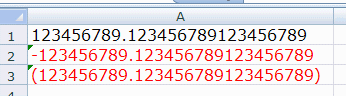## Formatting Thousands Separators

By default, xlPrecision does not add thousands separators such as the comma in "1,000". On all editions except for the 25 SD edition, you can optionally add them:

• In cell C4, change the formula "=xlpSUBTRACT(A1,A2,2)" to "=xlpSUBTRACT(A1,A2,2,TRUE)".

Note that the return value changes from "(86419753208641975320)" to "(86,419,753,208,641,975,320)".

The 4th argument is "format_thousands". format_thousands is ignored in the 25 SD and 35 SD editions, which never return thousands separators.

The thousands separator generated by format_thousands is internationalized. This means that the local thousands separator will be used -- for example, a comma in the USA, a period in Germany, and a space in France. Note, the decimal symbol is also internationalized -- for example, a period in the USA, a comma in Germany and France.

Results formatted with thousands separators can still be used as operands in other xlPrecision formulas.

Some xlPrecision functions use different syntax than this for formatting thousands separators. See the Function Reference entries for each xlPrecision function.

## Formatting Currency

On all editions except for the 25 SD edition, results can optionally be formatted with the local currency symbol (for example, "\$" in the USA):

• In cell C4, change the formula "=xlpSUBTRACT(A1,A2,2,TRUE)" to "=xlpSUBTRACT(A1,A2,2,TRUE,TRUE)".

Note that the return value changes from "(86,419,753,208,641,975,320)" to "(\$86,419,753,208,641,975,320)".

The 5th argument is "format_currency". format_currency is ignored in the 25 SD edition.

Results formatted with the local currency symbol can still be used as operands in other xlPrecision formulas.

Some xlPrecision functions use different syntax than this for formatting currency. See the Function Reference entries for each xlPrecision function.

## Formatting in Exponential Notation

On all editions except for the 25 SD edition, results can optionally be formatted in exponential notation:

• In cell C4, change the formula "=xlpSUBTRACT(A1,A2,2,TRUE,TRUE)" to "=xlpSUBTRACT(A1,A2,2,TRUE,TRUE,TRUE)".

Note that the return value changes from "(\$86,419,753,208,641,975,320)" to "(\$8.641975320864197532E+19)".

The 6th argument is "exponential_notation". exponential_notation is ignored in the 25 SD edition.

Results formatted with exponential notation can still be used as operands in other xlPrecision formulas.

Some xlPrecision functions use different syntax than this for formatting exponential notation. See the Function Reference entries for each xlPrecision function.

## Formatting Decimal Place

On the 5,000 SD edition and higher, and on the Free Edition, results can optionally be formatted to any decimal place you specify.

• In cell C4, change the formula to "=xlpSUBTRACT(A1,A2,,,,,,,3)".

Note that the return value changes to "86,419,753,208,641,975,320.000".

The 9th argument is "format_decimal_place". format_decimal_place is ignored in the 25 SD and 1,500 SD editions.

format_decimal_place was added in version 3.1, and currently applies only to the arithmetic operator functions, and to xlpFORMAT.

## Getting Faster Calculations

If you don't specify how many significant digits to return, you'll get 100, or less if your edition of xlPrecision only allows fewer than that. You can get faster calculations by specifying a lower number of significant digits. For example, this formula specifies 50 significant digits:

=xlpDIVIDE(2,3,,,,,50)

## Displaying & Printing Results Longer Than 1,024 Characters

Microsoft Excel 97 and later allows up to 32,767 text characters in a cell, and Excel 2007 and later can display and print all of them.

However, Excel normally displays only the first 1,024 characters in a cell. To view an xlPrecision return value longer than 1,024 characters, turn on "Wrap Text" for the cell:

• To turn on "Wrap Text" for the cell, right-click the cell and choose Format Cells. Then Select the Alignment tab, and turn on Wrap Text.

After turning on Wrap Text, you'll probably also need to widen the column and make the row height taller, and maybe make the font size smaller.

However, although that will display more than 1,024 characters, in Excel versions earlier than 2007 it won't display 32,767 characters. The exact number of characters it will display varies depending on your configuration. To view all 32,767 characters, you can do any of the following:

• Copy the cell, and Paste Special | Values to another cell. Then, with the new cell selected, look in the formula bar. The formula bar displays the entire result (up to 32,767 characters), though you may need to scroll down within the formula bar to see it all.

• Copy the cell and paste into Notepad or a word processor.

• Save the file as a text file type (Save As | Save as type = "text (tab delimited)"), and view the text file in Notepad or a Word processor.

• Using the Text Box tool on Excel's Drawing toolbar, draw a textbox on the worksheet. Select the cell and Copy | Paste Special | Values to another cell. With the new cell selected, click in the formula bar and select the entire contents. Copy the contents, and paste into the textbox.

Also, in Excel 2007 and later, you can view up to 8,192 characters in the formula bar by selecting the formula in the formula bar, and pressing F9 (but don't press Enter!). When done, be sure to press Escape to bring back the formula. If you inadvertently press Enter, try Undo (Ctrl + z) to bring back the formula.

To display and print all 32,767 characters in a cell in Excel 2007, you'll need to make the font size very tiny, in addition to maximizing the column width and row height. In some cases you may prefer some of the above workarounds even in Excel 2007.

Although Excel normally displays and prints only the first 1,024 characters in the cell, it makes all the characters (up to 32,767 characters) available to formulas in other cells, including xlPrecision formulas. The display of only 1,024 characters does not indicate any loss of precision in xlPrecision formulas.

## 32,767 SD Edition: Special Considerations

The 32,767 SD edition is only able to return 32,767 significant digits when the result is a positive integer not formatted with either thousands separators or currency symbols. This is because Excel only allows a maximum of 32,767 characters in a cell. If one of those characters is a decimal, then only 32,766 characters are left over for digits. The same is true of currency symbols and negative formatting. If the number is smaller than 1, then zeroes between the decimal and the first significant digit will also reduce the available number of significant digits. And of course, thousands separators can reduce it by up to 25%.

In all cases, the 32,767 SD edition will give you as many significant digits as possible with the formatting you have chosen.

If the return value is so large that it has more than 32,767 characters to the left of the decimal, then xlPrecision is of course unable to return a correct value and instead returns "#VALUE!". Note, that's a vastly larger number than Excel can return without xlPrecision. Excel itself can only return or recognize a number with a maximum of 308 digits to the left of the decimal.

## Insert Function Dialog

If you are using the 1,500 SD or higher edition, or the Free Edition, of xlPrecision, you can use Excel's Insert Function dialog to insert xlPrecision functions more conveniently than typing them in directly:

• Select the worksheet cell where you want the xlPrecision formula.
• Click the little "fx" to the left of Excel's formula bar. Or, click Formulas | Insert Function (in Excel 2003 and earlier, choose Insert | Function). The Insert Function dialog should appear.
• In the Insert Function dialog, bring down the Category dropdown, and select any of the High-Precision categories at the bottom of the list. The function list should show the xlPrecision functions in that category.
• Select a function and click OK. The Function Arguments dialog should appear for that function.
• For each of the argument fields, you can either type the argument in directly, or click the little square to the right of it, select a cell where you've put the desired argument. When you're done, click OK.
• If at any time you want to modify an existing xlPrecision formula, simply select that cell and click the little "fx" to the left of Excel's formula bar or choose Insert | Function. Instead of the Insert Function dialog, you'll then get the Function Arguments dialog, already filled out with the arguments you've already entered. Then, simply change them as desired and click OK.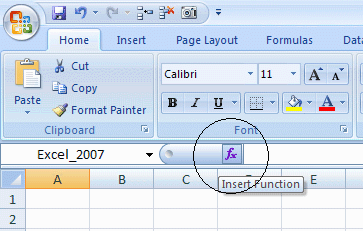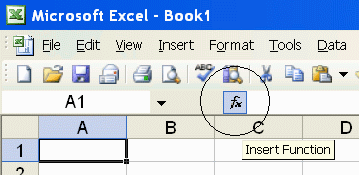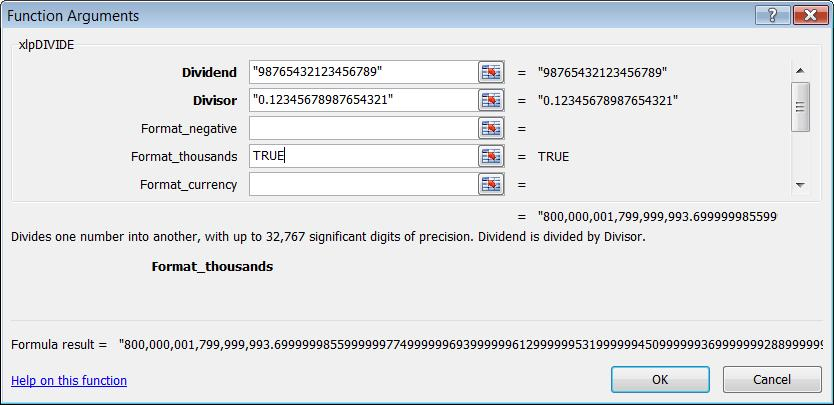Using the Insert Function dialog with xlPrecision functions requires the 1,500 SD or higher edition, or the Free Edition, of xlPrecision. Not recommended with the Free Edition as it causes the Free Edition dialog to appear multiple times.

 Using Excel's Insert Function Dialog with the xlPrecision Free Edition Using Excel's Insert Function dialog with the xlPrecision Free Edition is not recommended because it calculates on every keystroke, causing the Free Edition dialog to appear many times more than usual. Although it is not recommended in the Free Edition, it is enabled in the Free Edition so that you can evaluate it. If you do use it in the Free Edition, you might want to use Notepad to copy/paste whole arguments instead of typing them in, to minimize the number of times the Free Edition dialog appears.

Using Excel's Insert Function Wizard can be slow because Excel recalculates the function every time a digit or character is entered in the Wizard. To speed up that process, enter "0" for all numeric operands (such as num and rt for xlpROOT), so that the function does not take long to calculate. Then, when done with the Wizard, manually change the numeric operands to the desired numbers directly in the worksheet. Alternatively, you could use Notepad to copy/paste whole arguments instead of typing them in.

## Factorials

An interesting way to compare xlPrecision with Excel is to look at how they calculate factorials. A factorial is the number resulting from multiplying a whole number by every whole number between itself and 1, inclusive. For example, the factorial of 5 is:

1 x 2 x 3 x 4 x 5 = 120

Factorials are symbolized with an exclamation point ("!"). For example, the factorial of 5 is represented as "5!". An example of how factorials are used is to determine the total possible number of ways a given number of items can be arranged in a row. For example, the factorial of a standard deck of playing cards is 52!.

Without xlPrecision, Excel can calculate factorials up to 170!. But it can only calculate factorials at full precision up to 20! -- after that, Excel rounds them to the first 15 digits. xlPrecision can calculate factorials up to 9,273! -- all at full precision.

To see it for yourself:

• Create a new workbook in Excel.

• In cell A1, enter "=ROW()" (without quotes).

• Copy cell A1 down through A200.

• In cells B1 and C1, enter "1" (without quotes).

• In cell B2, enter "=xlpMULTIPLY(B1,A2)" (without quotes).

• In cell C2, enter "=C1*A2" (without quotes).

• Copy cells B2 and C2 down to B200 and C200. (But note, if you're using the Free Edition, you'll need to click OK once per row, so you might want to start out with fewer rows.)

• Select column C, and choose Format | Cells | Number tab. In the Category dropdown listbox, select Number. In the Decimal Places spinner, select 0.

• Widen columns B and C so that you can see as much of them both together as possible.

• Note that on rows 1 - 20, Excel (column C) and xlPrecision (column B) are identical.

• Note that cells B21 and C21 are different. In C21, excel has rounded it down. That's because 21! has 16 non-zero digits, and Excel can only give you the first 15 of them. xlPrecision, in cell B21, gives you all 16 non-zero digits.

• Note that at row 171, Excel (column C) starts returning "#NUM!". That's because Excel can't recognize a number that large.

• xlPrecision can return factorials down through row 9,273, though the precision depends on the xlPrecision edition. The 32,767 SD edition can return 9,273! at full precision, and the 1,500 SD edition can return 693! at full precision. The 35 SD and 25 SD editions can return 36! and 29! respectively at full precision, which may not sound like much but are still better than Excel's 20! at full precision.

• Getting back to the deck of playing cards (52!), Excel (cell C52), rounding 52! to 15 significant digits, returns:

80,658,175,170,943,900,000,000,000,000,000,000,000,000,000,000,000,000,000,000,000,000,000

• xlPrecision (cell B52), returning 52! at full precision, returns:

80,658,175,170,943,878,571,660,636,856,403,766,975,289,505,440,883,277,824,000,000,000,000

(That number is precisely correct -- the final 12 zeroes are not due to rounding.)

• That's a difference of this many possible arrangements of playing cards!

21,428,339,363,143,596,233,024,710,494,559,116,722,176,000,000,000,000

## Data Control & Analysis Features

xlPrecision offers many additional features that take you beyond Excel's capabilities in other ways. See Data Control & Analysis Features.

Find xlPrecision's online help by choosing Add-Ins > xlPrecision 3.1 > xlPrecision 3.1 Help and Function Reference: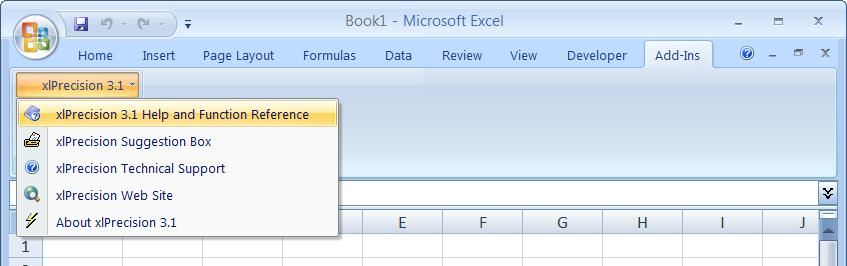In Excel 2003 and earlier, xlPrecision's online help is in Tools > xlPrecision 3.1 > xlPrecision 3.1 Help and Function Reference:For helpful tips on using xlPrecision, see:

Tips

For more detail on usage and syntax, please see:

Function Reference

For help on troubleshooting common problems, see:

Troubleshooting F.A.Q.